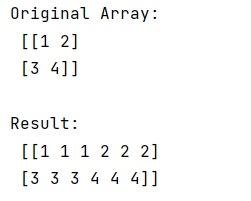# numpy.repeat() Method with Example

Python | numpy.repeat(): Learn about the numpy.repeat() method, its usages and example.
Submitted by Pranit Sharma, on February 27, 2023

NumPy is an abbreviated form of Numerical Python. It is used for different types of scientific operations in python. Numpy is a vast library in python which is used for almost every kind of scientific or mathematical operation. It is itself an array which is a collection of various methods and functions for processing the arrays.

## numpy.repeat() Method

The numpy.repeat() is a simple function in numpy that is used to repeat elements in a numpy array.

It takes an input array, the number of repetitions, and the axis along which the elements must be repeated.

It returns an output array of repeated elements along the specified axis.

Syntax:

```numpy.repeat(a, repeats, axis=None)
```

Parameter(s):

• a: input array
• repeats: number of repetitions.
• axis: axis of array along which repetition must be applied.

Let us understand with the help of an example,

## Python code to demonstrate the example of numpy.repeat() method

```# Import numpy
import numpy as np

# Creating a numpy array
arr = np.array([[1,2],[3,4]])

# Display original array
print("Original Array:\n",arr,"\n")

# Repeating elements of array
# 3 times along the column
res = np.repeat(arr,3,axis=1)

# Display result
print("Result:\n",res)
```

Output:Languages: » C » C++ » C++ STL » Java » Data Structure » C#.Net » Android » Kotlin » SQL
Web Technologies: » PHP » Python » JavaScript » CSS » Ajax » Node.js » Web programming/HTML
Solved programs: » C » C++ » DS » Java » C#
Aptitude que. & ans.: » C » C++ » Java » DBMS
Interview que. & ans.: » C » Embedded C » Java » SEO » HR
CS Subjects: » CS Basics » O.S. » Networks » DBMS » Embedded Systems » Cloud Computing
» Machine learning » CS Organizations » Linux » DOS
More: » Articles » Puzzles » News/Updates